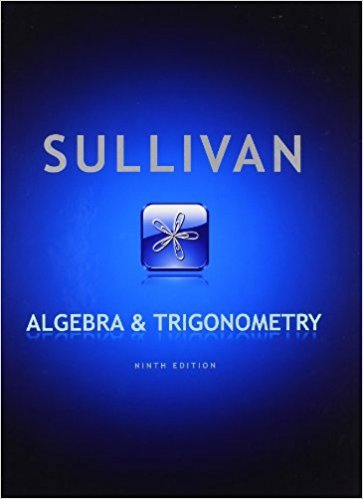×
×

# Solutions for Chapter 4.1: Algebra and Trigonometry 9th Edition## Full solutions for Algebra and Trigonometry | 9th Edition

ISBN: 9780321716569Solutions for Chapter 4.1

Solutions for Chapter 4.1
4 5 0 398 Reviews
30
5
##### ISBN: 9780321716569

Since 56 problems in chapter 4.1 have been answered, more than 58154 students have viewed full step-by-step solutions from this chapter. This textbook survival guide was created for the textbook: Algebra and Trigonometry, edition: 9. Algebra and Trigonometry was written by and is associated to the ISBN: 9780321716569. This expansive textbook survival guide covers the following chapters and their solutions. Chapter 4.1 includes 56 full step-by-step solutions.

Key Math Terms and definitions covered in this textbook
• Block matrix.

A matrix can be partitioned into matrix blocks, by cuts between rows and/or between columns. Block multiplication ofAB is allowed if the block shapes permit.

• Column space C (A) =

space of all combinations of the columns of A.

• Complex conjugate

z = a - ib for any complex number z = a + ib. Then zz = Iz12.

• Cross product u xv in R3:

Vector perpendicular to u and v, length Ilullllvlll sin el = area of parallelogram, u x v = "determinant" of [i j k; UI U2 U3; VI V2 V3].

• Distributive Law

A(B + C) = AB + AC. Add then multiply, or mUltiply then add.

• Four Fundamental Subspaces C (A), N (A), C (AT), N (AT).

Use AT for complex A.

• Fourier matrix F.

Entries Fjk = e21Cijk/n give orthogonal columns FT F = nI. Then y = Fe is the (inverse) Discrete Fourier Transform Y j = L cke21Cijk/n.

• Full column rank r = n.

Independent columns, N(A) = {O}, no free variables.

• Hermitian matrix A H = AT = A.

Complex analog a j i = aU of a symmetric matrix.

• Indefinite matrix.

A symmetric matrix with eigenvalues of both signs (+ and - ).

• Iterative method.

A sequence of steps intended to approach the desired solution.

• Multiplier eij.

The pivot row j is multiplied by eij and subtracted from row i to eliminate the i, j entry: eij = (entry to eliminate) / (jth pivot).

• Network.

A directed graph that has constants Cl, ... , Cm associated with the edges.

• Outer product uv T

= column times row = rank one matrix.

• Pseudoinverse A+ (Moore-Penrose inverse).

The n by m matrix that "inverts" A from column space back to row space, with N(A+) = N(AT). A+ A and AA+ are the projection matrices onto the row space and column space. Rank(A +) = rank(A).

• Random matrix rand(n) or randn(n).

MATLAB creates a matrix with random entries, uniformly distributed on [0 1] for rand and standard normal distribution for randn.

• Right inverse A+.

If A has full row rank m, then A+ = AT(AAT)-l has AA+ = 1m.

• Rotation matrix

R = [~ CS ] rotates the plane by () and R- 1 = RT rotates back by -(). Eigenvalues are eiO and e-iO , eigenvectors are (1, ±i). c, s = cos (), sin ().

• Solvable system Ax = b.

The right side b is in the column space of A.

• Vector space V.

Set of vectors such that all combinations cv + d w remain within V. Eight required rules are given in Section 3.1 for scalars c, d and vectors v, w.

×